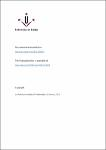## Cyclicity of a class of polynomial nilpotent center singularitiesIn this work we extend techniques based on computational algebra for bounding the cyclicity of nondegenerate centers to nilpotent centers in a natural class of polynomial systems, those of the form $\dot x = y + P_{2m + 1}(x,y)$, $\dot y = Q_{2m + 1}(x,y)$, where $P_{2m+1}$ and $Q_{2m+1}$ are homogeneous polynomials of degree $2m + 1$ in $x$ and $y$. We use the method to obtain an upper bound (which is sharp in this case) on the cyclicity of all centers in the cubic family and all centers in a broad subclass in the quintic family.